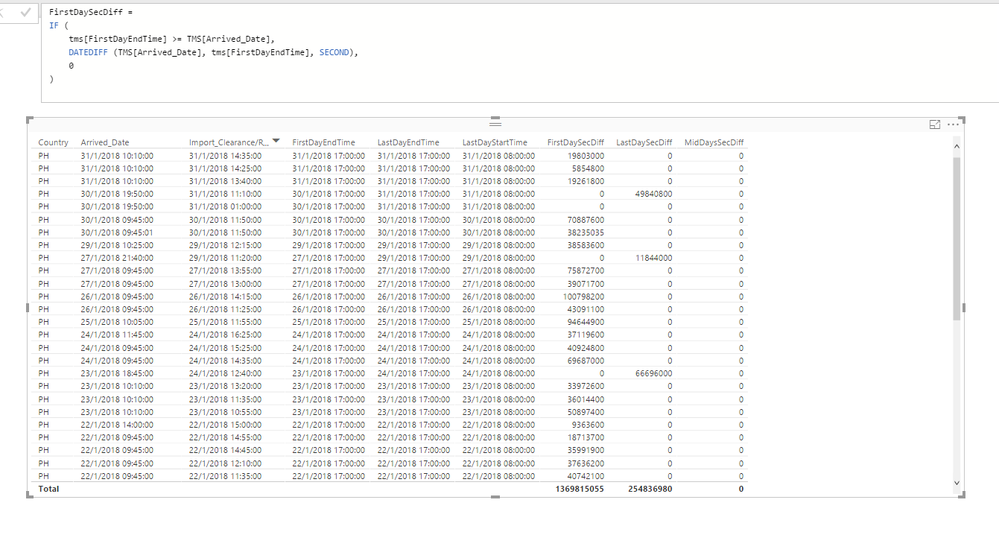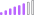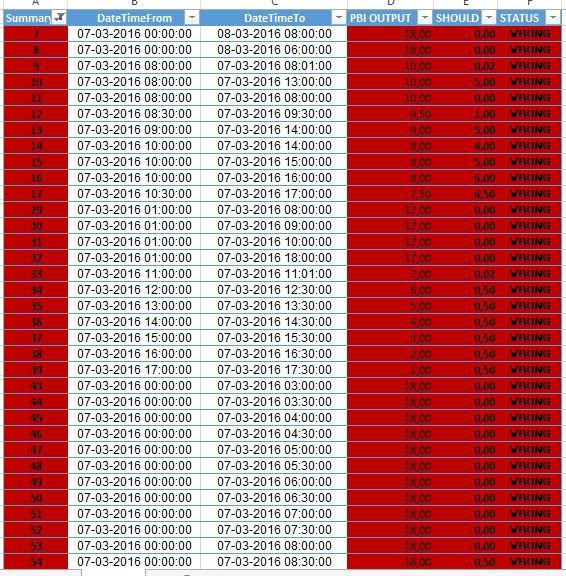cancel
Showing results for
Did you mean:## Calculate Date and Time difference considering the weekends and workhours

Hi everybody!

I'm still learning how to use Power BI, I search for this everywhere but I didn't found an answer!

Here is the thing,

I need to calculate the difference between dates and time, but the thing is that I need to calculate just the working days and the workhours.Taking the first line as an example: (03/11/2016 13:57:22 - 03/01/201613:36:38) the column hours should be 63:38:38

Considering that 03/05 and 06/05 are saturday and sunday, and considering that the work hours are from 08:00AM to 18:00PM.

How can I solve that!

Thank you!

2 ACCEPTED SOLUTIONSMicrosoft

@dcs136

The only possibility I can think of is that there is something wrong with the Calendar'[WorkDay] formula. Could you please check this column formula is like below? The data type of this column should be “True/False”.

If it is actually same as mine, could you please upload your .pbix file to OneDrive and share it with me? In that case I can take a look at your .pbix file and try to solve the problem.Best Regards,

HerbertMicrosoft

@dcs136

How about the result if we update the “FirstDaySecDiff” measure as below?

```FirstDaySecDiff =
IF (
Table1[FirstDayEndTime] >= Table1[DateTimeFrom]
&& Table1[FirstDayEndTime] <= Table1[DateTimeTo],
DATEDIFF ( Table1[DateTimeFrom], Table1[FirstDayEndTime], SECOND ),
IF (
Table1[FirstDayEndTime] >= Table1[DateTimeFrom]
&& Table1[FirstDayEndTime] > Table1[DateTimeTo],
DATEDIFF ( Table1[DateTimeFrom], Table1[DateTimeTo], SECOND ),
0
)
)```

Best Regards,

Herbert

43 REPLIES 43Frequent Visitor

Hi everyone, im recently working with power bi and dax, i have just been asked to do this calculation and im not very clear on how to implement the code, i think that understanding the model would help me, could someone help me please?.

regards,Frequent Visitor

Really appreciate the work y'all put in to get a working DAX solution. I turned it into a custom M function so that you can easily apply it to multiple columns without having a ton of duplication. Also fixed a couple of small bugs that were in the original DAX.

To use, just copy this into a new blank query and should work out of the box.

Of course you're free to change the start/end times (currently at 8am and 5pm) etc

```(from, to) => let
// utility functions
dateWithSetTime = (dt, t) => DateTime.From(Date.ToText(Date.From(dt)) & " " & Time.ToText(t)),
max2 = (first, second) => if first > second then first else second,
min2 = (first, second) => if first < second then first else second,
isBetween = (t, first, last) => t >= first and t <= last,
isWeekend = (day) => Date.DayOfWeek(day) = 5 or Date.DayOfWeek(day) = 6,
isWorkday = (day) => not isWeekend(day),
numDaysBetween = (f, t) =>
let
length = Duration.Days(Date.From(to) - Date.From(from)) - 1,
dates = List.Dates(start, length, #duration(1, 0, 0, 0)),
workdays = List.Select(dates, each isWorkday(_))
in
List.Count(workdays),
dur = (days) => Duration.From(days),

// variables and initial setup
startTime = #time(8,0,0),
endTime = #time(17,0,0),
firstDayStartTime = dateWithSetTime(from, startTime),
firstDayEndTime = dateWithSetTime(from, endTime),
lastDayStartTime = dateWithSetTime(to, startTime),
lastDayEndTime = dateWithSetTime(to, endTime),
endsOnStartDay = Date.From(from) = Date.From(to),

// get seconds for first, middle, and last days
firstDaySeconds =
// make sure that end date is after start date
if from > to then error "The start date is greater than the end date. Make sure that you passed the arguments in the right order." else
// record 0 time if weekend, starts after day ends, or ends before day starts
if isWeekend(Date.From(from)) or from > firstDayEndTime or to < firstDayStartTime
then dur(0)
else min2( to, firstDayEndTime ) - max2( from, firstDayStartTime ),

middleDaysSeconds =
if endsOnStartDay
then dur(0)
else (endTime - startTime) * numDaysBetween(from, to),

lastDaysSeconds =
// record 0 time if holiday, weekend, already counted, or ends before day starts
if isWeekend(Date.From(to)) or endsOnStartDay or to < lastDayStartTime
then dur(0)
else min2(to, lastDayEndTime) - lastDayStartTime,

totalSeconds = Duration.TotalSeconds(firstDaySeconds + middleDaysSeconds + lastDaysSeconds)
in
totalSeconds / 3600```

Pro tip: enable M syntax highlighting in Preview features options, it makes it so much more pleasant to write in.Anonymous
Not applicable

@sam_woolerton this is fantastic, thank you so much! I did have to make one small change to get the hours to calculate properly - using the default Date.DayofWeek settings, the weekends are days 0 and 6 rather than 5 and 6.Frequent Visitor

Sam,

I am actually running into an issue on some of the output.

FirstStartDateTime: 10/7/2017 1:20:00 PM

CreateDate: 10/10/2017 8:39:18 AM

An error occurred in the ‘’ query. Expression.Error: The 'increment' argument is out of range.
Details:
1.00:00:00

I'm not sure what is happening here. I kept everything the same, and also changed the startTime, but am getting the same error. Any ideas?Frequent Visitor

@itshudak looks like you passed the arguments in the wrong order (doing that reproduced the exact error for me).

I've updated my code above to give a helpful error message in that caseFrequent Visitor

Reversing the inputs actually gives me the same error, but 10 times worse. The output should be positive, correct? Regardless, I don't know what this error is actually saying, but my data looks correct, so I don't think it's a formatting issue. I'm just confused as to what needs to be fixed to get it to work properly. I have 100k rows that work, but these 100 or so errors have got me for a loop.Frequent Visitor

@itshudak can you put together a reproducible example? Check this out for pointers

Easiest way would be to put together a CSV with 2 columns (start and end time) and 5-10 rows, made up from your problem rows. Import this into Power BI and make sure you still get the error

I'll then check it out and see what I can doFrequent Visitor

Nevermind, consider this egg on my face. It was actually an issue with the stop time being before the start time and I was just getting mixed up. Thank you for your help with this, I appreciate it.New Member

@dcs136 can you also look at my problem? have you occured this before?New Member

Hello Herbert,

I am looking at  your solution, but can you have a look at my data? The FirstDaySecDiff, LastDaySecDiff  and MidDaysSecDiff are too large to be normal. I have no idea where is wrong. Thanks!!!Frequent Visitor

Could someone help me find the error in this expression? I get blank values where there should be a count, but the 0s work for time differences on the same day.

MidDaysSecDiff =
IF (Table1[DateTimeFrom].[Date] <> Table1[DateTimeTo].[Date],
CALCULATE( DISTINCTCOUNT('Calendar2'[Date]), FILTER('Calendar2','Calendar2'[Date] > Table1[FirstDayEndTime] && 'Calendar2'[Date] < Table1[LastDayStartTime] && 'Calendar2'[WorkDay2] = TRUE())),0)Microsoft

@dcs136

There may be several methods to get the expected result. I’ll divide the difference to three parts, the first day, the middle days and the last day. For details, please refer to following steps.

I’ve also upload my .pbix file here for reference.

1. Create a calendar table with following formula. But do not create relationship between these two tables.
```Calendar =
CALENDAR ( "1/1/2016", "12/31/2016" )```
2. Create a column in calendar table to mark the working days.
```WorkDay =
VAR WeekDayNum =
WEEKDAY ( 'Calendar'[Date], 2 )
RETURN
(
IF ( WeekDayNum = 6 || WeekDayNum = 7, FALSE (), TRUE () )
)```
3. Create a column to store the working end time of first day.
```FirstDayEndTime =
DATE ( YEAR ( Table1[DateTimeFrom] ), MONTH ( Table1[DateTimeFrom] ), DAY ( Table1[DateTimeFrom] ) )
& " 18:00:00"```
4. Create a column to calculate the working seconds of first day.
```FirstDaySecDiff =
DATEDIFF ( Table1[DateTimeFrom], Table1[FirstDayEndTime], SECOND )```
5. Create a column to store the working start time of last day.
```LastDayStartTime =
DATE ( YEAR ( Table1[DateTimeTo] ), MONTH ( Table1[DateTimeTo] ), DAY ( Table1[DateTimeTo] ) )
& " 8:00:00"```
6. Create a column to store the working end time of last day.
```LastDayEndTime =
DATE ( YEAR ( Table1[DateTimeTo] ), MONTH ( Table1[DateTimeTo] ), DAY ( Table1[DateTimeTo] ) )
& " 18:00:00"```
7. Create a column to calculate the working seconds of last day.
```LastDaySecDiff =
IF (
FORMAT ( Table1[DateTimeFrom], "Short Date" )
<> FORMAT ( Table1[DateTimeTo], "Short Date" ),
IF (
Table1[DateTimeTo] >= Table1[LastDayStartTime]
&& Table1[DateTimeTo] <= Table1[LastDayEndTime],
DATEDIFF ( Table1[LastDayStartTime], Table1[DateTimeTo], SECOND ),
IF (
Table1[DateTimeTo] > Table1[LastDayEndTime],
DATEDIFF ( Table1[LastDayStartTime], Table1[LastDayEndTime], SECOND ),
0
)
),
0
)```
8. Create a column to calculate the working seconds of middle days.
```MidDaysSecDiff =
IF (
FORMAT ( Table1[DateTimeFrom], "Short Date" )
<> FORMAT ( Table1[DateTimeTo], "Short Date" ),
3600 * 10
* (
CALCULATE (
DISTINCTCOUNT ( 'Calendar'[Date] ),
FILTER (
'Calendar',
'Calendar'[Date] > Table1[FirstDayEndTime]
&& 'Calendar'[Date] < Table1[LastDayStartTime]
&& 'Calendar'[WorkDay] = TRUE ()
)
)
- 1
),
0
)```
9. Create the final column to calculate the total working hours.
```TotalHourDiff =
( Table1[FirstDaySecDiff] + Table1[LastDaySecDiff]
+ Table1[MidDaysSecDiff] )
/ 3600```

Note: The data type of columns of “FirstDayEndTime”, “LastDayStartTime” and “LastDayEndTime” should be Date/Time as below.Best Regards,

HerbertNew Member

Hi!

I think there is a mistake in your solution.

Formula for FirstDaySecDiff is only correct if DateTimeFrom's date is different from DateTimeTo's date

```FirstDaySecDiff =
DATEDIFF ( Table1[DateTimeFrom], Table1[FirstDayEndTime], SECOND )```

Otherwise the right formula is as follows:

`FirstDaySecDiff = IF (FORMAT (Table1[DateTimeFrom], “Short Time”) = FORMAT (Table1[DateTimeTo], “Short Time”),DATEDIFF ( Table1[DateTimeFrom], Table1[DateTimeTo], SECOND ),DATEDIFF ( Table1[DateTimeFrom], Table1[FirstDayEndTime], SECOND ))`

I tried with this new formula and now i get right calculation results.

Best regards,

AndrésFrequent Visitor

If it is helpful, I believe I found a solution that can be implemented without using reference tables. It also applies to business hours Monday to Friday only :

https://community.powerbi.com/t5/Desktop/Calculating-Working-hours/m-p/1233459/highlight/true#M54659...Helper III

wonder if anyone could help?  I have the following:

MidDaysSecDiff =
IF (
FORMAT ( msdyn_audithistories[Start time], "Short Date" )
<> FORMAT ( msdyn_audithistories[createdon], "Short Date" ),
3600 * 10
* (
CALCULATE (
DISTINCTCOUNT ( 'Calender'[Date] ),
FILTER (
'Calender',
'Calender'[Date] > msdyn_audithistories[FirstDayEndTime]
&& 'Calender'[Date] < msdyn_audithistories[LastDayStartTime]
&& 'Calender'[WorkDay] = TRUE ()
)
)
- 1
),
0
)

However i need to state that if the msdyn_audithistories[Start time] ie the start time is blank that it returns 0? any pointers?

Many thanksPost Patron

This solution present errors in the following cases!

Can sombody share a solution??? (the pbix and the excel data source will be available here):

https://www.dropbox.com/s/5b4zmo40k9dsiwl/time%20difference%20considering%20only%20friday%20to%20mon...Helper III

thank you for contributing, this is excately what i was looking for.  One quick question, we have different opening and closing hours for certain customers, do anyone have any suggestions on how i could overcome this issue:

ie customer A is 09:00 to 17:00

Customer b is 08:30 to 17:30 etc etc

Any help would be very much appriciatedHerbert,

Thank you so much for your help.

But unfortunately DATEDIFF  gives an error "In DATEDIFF function, the start date cannot be greater than the end date"

because some hours on "DateTimeFrom" are greater than the "FirstDayEndTime" as you can see on the picture

I tried to fix this, but I couldn't do it.

Thank you!Microsoft

@dcs136

Please update the DAX formula of FirstDaySecDiff column as below and have a try again.

```FirstDaySecDiff =
IF (
Table1[FirstDayEndTime] >= Table1[DateTimeFrom],
DATEDIFF ( Table1[DateTimeFrom], Table1[FirstDayEndTime], SECOND ),
0
)```

Best Regards,

HerbertHelper I

is there a way i can do a reversal on the same thing that you explained above - I have a date table and i am able to calculate working days.(0s for weekends and 1's for Weekdays). I need to add 5 days to my start date and and pick the appropriate working date from the date table so that it gives me an "Expected Completion Date" that takes account of weekends.